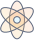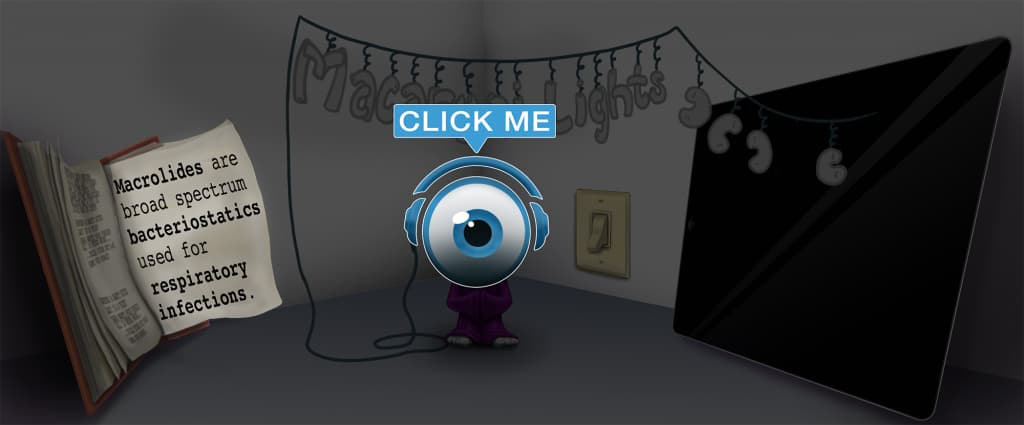# Master IV Calculation Formulas with Picmonic for Nursing RN

## With Picmonic, facts become pictures. We've taken what the science shows - image mnemonics work - but we've boosted the effectiveness by building and associating memorable characters, interesting audio stories, and built-in quizzing.

### IV Calculation Formulas

IV CalculatorPicmonic
With orders for IV fluid administration, it is the nurse’s responsibility to make sure the fluid will infuse at the prescribed rate. The drop factor is the number of drops in one mL of solution, and this will be printed on the IV tubing package. Macrodrip and microdrip refer to the diameter of the needle where the drop enters the drip chamber. The drop factor is needed in order to calculate the drops per minute. In general, a standard set is called a macrodrip, and it generally has a drip factor of 10, 12, 15, or 20 gtt/ml (drops per milliliter). Macrodrips are used for general adult IV administrations. A microdrip set is also called a minidrip, and it is 60 gtt/ml. Microdrips are usually used when more exact measurements are required, such as for pediatric patients or ICU patients. The calculation for flow rate is: total volume to infuse (in mL) x drop factor/ time in minutes = drops/minute (gtt/min). The calculation for infusion time is: total volume to infuse/milliliter per hour being infused = infusion time. The calculation for the number of milliliters per hour is: total volume in milliliters /time in hours = number of milliliters/hour.
6 KEY FACTS
IV TUBING
Drop Factor
Drop Factory

The drop factor is the number of drops in one mL of solution, and this will be printed on the IV tubing package. Macrodrip and microdrip refer to the diameter of the needle where the drop enters the drip chamber. The drop factor is needed in order to calculate the drops per minute.

Macrodrip
Macaroni-drop

In general, a standard set is called a macrodrip, and it generally has a drop factor of 10, 12, 15, or 20 gtt/ml (drops per milliliter). Macrodrips are used for general adult IV administrations.

Microdrip
Microphone-drop

A microdrip set is also called a minidrip, and it is 60 gtt/ml. Microdrips are usually used when more exact measurements are required, such as for pediatric patients or ICU patients.

FLOW RATES
Total Volume to Infuse (mL) X Drop Factor/ Time in Minutes = Drops/Minute (gtt/min)
Total Volume-cup X Drop Factory / Minute-timer = Drops per Minute-timer

The formula for calculating the drops per minute (gtt/min) is the total volume to infuse (in mL),multiplied by the drop factor, divided by time in minutes, which equals the drops per minute (gtt/min).

INFUSION TIME
Total Volume to Infuse / mL per Hour Being Infused = Infusion Time
Total Volume-cup / Millionaire-liter-of-cola per Hourglass = In-fused-IV Clock

The formula for calculating the infusion time is the total volume to infuse divided by milliliters per hour being infused, which will give you the infusion time.

NUMBER OF ML/HR
Total Volume in mL / Time in Hours = Number of mL per Hour
Total Volume-cup in Millionaire / Hourglass = # of Millionaire-liter-of-cola and Hourglass

The formula for calculating the number of milliliters per hour is the total volume in milliliters divided by the time (in hours), which will result in the number of milliliters per hour.

Unlock all 6 facts & definitions with Picmonic Free!

JOIN FREE

## Take the IV Calculation Formulas Quiz

Picmonic's rapid review multiple-choice quiz allows you to assess your knowledge.

START QUIZ NOW

## Picmonic for Nursing RN CoversNursing RN
1,100+ Videos
13,000+ Facts

## Our Story Mnemonics Increase Mastery and Retention

Memorize facts with phonetic mnemonics

Unforgettable characters with concise but impactful videos (2-4 min each)

### Ace Your Nursing (RN) Classes & Exams with Picmonic:

#### Over 1,060,000 students use Picmonic’s picture mnemonics to improve knowledge, retention, and exam performance.